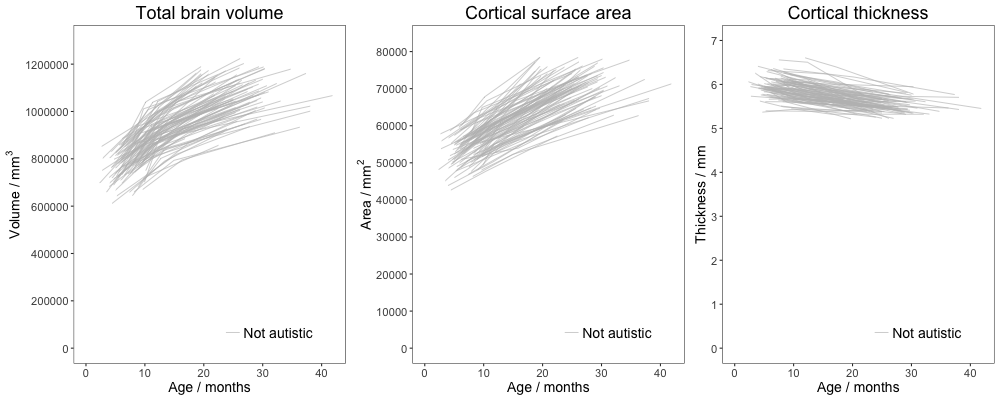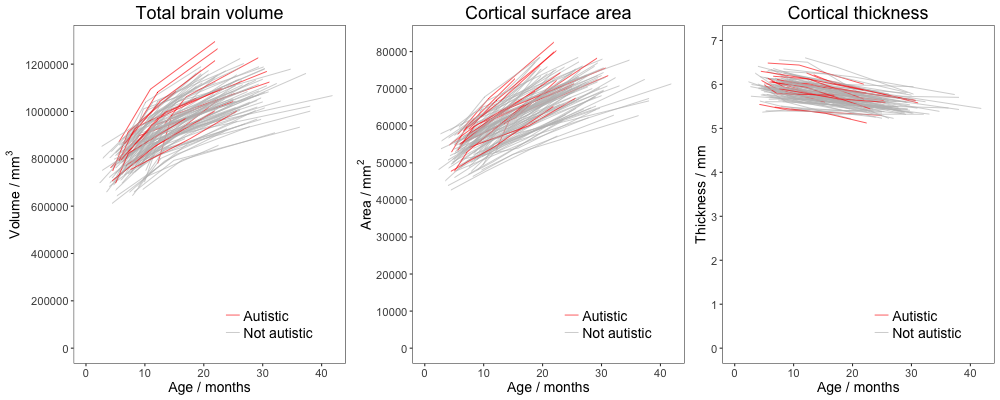# 1 Background

This accompanies my piece for the Head Quarters blog on The Guardian website: https://www.theguardian.com/science/head-quarters/2017/feb/23/autism-diagnosis-by-brain-scan-its-time-for-a-reality-check

The code plots data from Hazlett et (2017)’s study of brain growth in babies at high familial risk of autism.

In the first part I replot Figure 1 from the paper, which shows longitudinal growth curves. Animating the data shows more clearly the differences and overlap between babies who do vs do not receive an autism diagnosis.

The second part uses waffle plots to represent the outcome of machine learning techniques applied to the brain data to predict diagnostic outcome.

# 2 Libraries

``````library(openxlsx)
library(ggplot2)
library(grid)
library(magick)
library(knitr)
library(waffle)``````

# 3 Longitudinal growth curves

Underlying data for the plot were included in the online version of the paper. Read in excel file. Exclude the “low risk” group (coded as 0). Rename the two remaining groups.

``````Data <- read.xlsx("data/nature21369-f1.xlsx")
Data <- Data[(Data\$DSM3G!=0),]
Data\$DSM3G <- as.factor(Data\$DSM3G)
levels(Data\$DSM3G)[levels(Data\$DSM3G)=="1"] <- "Not autistic"
levels(Data\$DSM3G)[levels(Data\$DSM3G)=="2"] <- "Autistic"
Data\$TimePoint <- rep(c(6,12,24),length(unique(Data\$rCandID)))``````

## 3.2 Function: PlotFig

``````PlotFig <- function(Data, DV) {
myPlot <- ggplot(data=Data, aes_string(x = "Length_Age", y = DV, group = "rCandID", colour = "DSM3G")) +
geom_freqpoly(stat = "identity", size = I(.3)) +
scale_x_continuous("Age / months",limits=c(0,42),breaks=seq(0, 40, 10)) +
theme_bw() +
theme(text=element_text(size=14), plot.title = element_text(hjust = 0.5, size=18), legend.justification=c(1,0), legend.position=c(0.9,0.05), legend.title=element_blank(), legend.text = element_text(size = 14), axis.line = element_blank(), panel.grid.major = element_blank(), panel.grid.minor = element_blank(), panel.background = element_blank()) +
scale_colour_manual(values=c("grey", "red"), breaks=c("Autistic","Not autistic"))
myPlot
}   ``````

## 3.3 Function: PlotPanelFig

This function produces a three-panel figure showing total brain volume, surface are, and cortical depth. Individual plots are created in ggplot2 and combined using grid.arrange from gridExtra.

``````PlotPanelFig <- function(Data, FName = "Image.png") {

TBV <- PlotFig(Data=Data, DV="TBV") +
ggtitle("Total brain volume") +
scale_y_continuous(limits=c(0,1300000),breaks=seq(0, 1400000, 200000)) +
ylab(bquote('Volume / '*mm^3*''))

SA <- PlotFig(Data=Data, DV="SAAll") +
ggtitle("Cortical surface area") +
scale_y_continuous(limits=c(0,83000),breaks=seq(0, 80000, 10000)) +
ylab(bquote('Area / '*mm^2*''))

CT <- PlotFig(Data=Data, DV="CTAll") +
ggtitle("Cortical thickness") +
scale_y_continuous(limits=c(0,7),breaks=seq(0, 7, 1)) +
ylab("Thickness / mm")

# NB This method of combining plots ensures that all the plot areas are equal in size
plots <- list(TBV, SA, CT)
grobs <- lapply(plots, ggplotGrob)
g <- do.call(gridExtra::cbind.gtable, grobs)

png(filename=paste0("output/figures/",FName), width=1000, height=400)
grid.draw(g)
dev.off()
}  ``````

## 3.4 Generate animation frames

Save the two frames as PNG files. Frame 1 includes just the data from the babies who were not diagnosed with autism. Frame 2 includes both groups.

``PlotPanelFig (Data[(Data\$DSM3G=="Not autistic"),], FName="Frame1.png")``
``````## quartz_off_screen
##                 2``````
``knitr::include_graphics("output/figures/Frame1.png")````PlotPanelFig (Data, FName="Frame2.png")``
``````## quartz_off_screen
##                 2``````
``knitr::include_graphics("output/figures/Frame2.png")``## 3.5 Create GIF animation

Combine the two frames as a GIF. This requires the “magick” R package.

``````Frames <- c(image_read("output/figures/Frame1.png"), image_read("output/figures/Frame2.png"))
Animation <- image_animate(image_scale(Frames, "1000x400"), fps = 0.5, dispose = "previous")
image_write(Animation, "output/figures/BrainGrowth.gif")
knitr::include_graphics("output/figures/BrainGrowth.gif")``````